Refer to our Texas Go Math Grade 1 Answer Key Pdf to score good marks in the exams. Test yourself by practicing the problems from Texas Go Math Grade 1 Unit 3 Assessment Answer Key.

Vocabulary

Write to complete the related facts. (p. 460)

Question 1.
9 + 7 = 16
7 + 9 = 16

16 – 7 = 9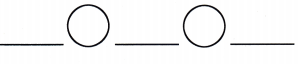Answer: 16 – 9 = 7Concepts and Skills

Complete the model and the number sentence.

Question 2.
Alii has 10 grapes. She eats 5 grapes. How many grapes does she still have? (TEKS 1.5.D)10 – 5 = _________Count forward by ones. Write the numbers. (TEKS 1.5.A)

Question 3.
35, 36, _______, _______, _______, _______
36 + 1 = 37
37 + 1 = 38
38 + 1 = 39
39 + 1 = 40
35, 36, 37, 38, 39, 40

Count backward by ones. Write the numbers. (TEKS 1.5.A)

Question 4.
96, 95, _______, _______, _______, _______
95 – 1 = 94
94 – 1 = 93
93 – 1 = 92
92 – 1 = 91
96, 95, 94, 93, 92, 91

Question 5.
There are 15 balloons. 8 balloons are green. The rest are purple. How many balloons are purple? (TEKS 1.5.D)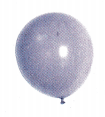(A) 7
(B) 15
(C) 8
Given,
There are 15 balloons. 8 balloons are green.
15 – 8 = 7
Thus the answer is option A.

Question 6.
Which is the related fact for 9 + 7 = 16? (TEKS 1.5.F, 1.5.G)
(A) 9 – 7 = 2
(B) 16 – 7 = 9
(C) 17 – 9 = 8
Answer: (B) 16 – 7 = 9

Question 7.
Which shows the same addends in a different order? (TEKS 1.5.F)
5 + 9 = 14
(A) 5 + 8 = 13
(B) 9 + 4 = 13
(C) 9 + 5 = 14
Answer: (C) 9 + 5 = 14

Question 8.
Which addition fact can you use to check the subtraction for 13 – 8 = _________? (TEKS 1.5.F, 1.5.G)
(A) 10 + _________ = 13
(B) 13 + _________ = 13
(C) 8 + _________ = 13
Answer: (C) 8 + _________ = 13

Question 9.
There are 3 turtles in the pond. Some more turtles join them. Now there are 9 turtles in the pond. How many turtles joined them? (TEKS 1.5.D)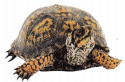(A) 11
(B) 6
(C) 12
There are 3 turtles in the pond. Some more turtles join them. Now there are 9 turtles in the pond.
9 – 3 = 6
6 turtiles joined them.
Thus the answer is option B.

Question 10.
There are 12 deer in the woods. Seven of the deer run away. Five deer are left in the woods. Which fact can you use to check the subtraction? (TEKS 1.5.F, 1.5.G)
(A) 6 + 9 = 15
(B) 12 + 5 = 17
(C) 5 + 7 = 12
Answer: (C) 5 + 7 = 12

Question 11.
Which is the unknown number? (TEKS 1.5.F, 1.5.G)
_________ + 8 = 17
17 – 8 = __________
(A) 17
(B) 9
(C) 3
_________ + 8 = 17
9 + 8 = 17
17 – 8 = 9
Thus the answer is option B.

Question 12.
Tamara has 3 dolls. Kelly has 7 dolls. How many fewer dolls does Tamara have than Kelly? (TEKS 1.5.D)(A) 3
(B) 10
(C) 4
Given,
Tamara has 3 dolls. Kelly has 7 dolls.
7 – 3 = 4
Thus the answer is option C.

Question 13.
Which number shows expressions of equal value? (TEKS 1.5.E)
6 + 3 = _________ – 2
(A) 10
(B) 6
(C) 11
11 – 2 = 9
Thus the answer is option C.

Question 14.
Amy has 7 gifts. Then she gets 2 more gifts. Then she gets 3 more gifts. How many gifts does Amy have? (TEKS 1.5.F)(A) 12
(B) 9
(C) 10
7 + 2 + 3 = 12
Thus the answer is option A.

Question 15.
Which number is ten less than 57? (TEKS 1.5.C)
(A) 75
(B) 47
(C) 40
57 – 10 = 47
Thus the answer is option B.

Question 16.
Use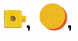or real objects to solve.
There are 15 children in the park. ________ children are playing ball. _______ children are not playing ball.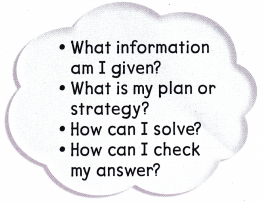• Write numbers to complete the story problem.
• Explain how you chose the numbers
• Write the related facts for the story problem.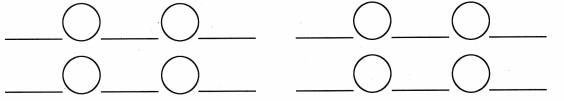• Explain how to use addition to check subtraction. (TEKS 1.3.D, 1.3.E, 1.5.G) Justify. Explain why your answer is reasonable.